# Physics - Electromagnetism - Capacitors (Condensers) and Capacitance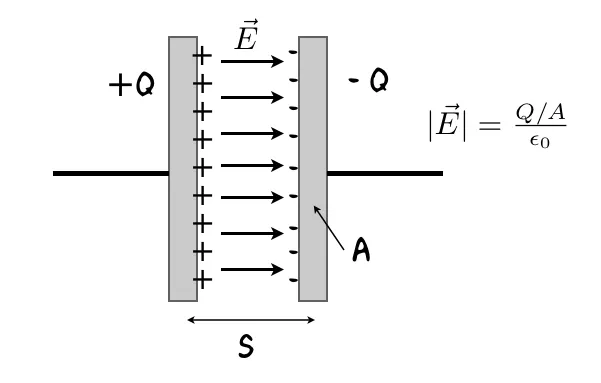Source

## Introduction

Hello it's a me again Drifter Programming!

Today we continue with Electromagnetism to get into Capacitors and Capacitance.

All the mathematical equations will be drawn using quicklatex!

So, without further do, let's get started!

## Capacitor (Condenser)

Until now we covered how electric force is applied to charged particles from the generated electric field around a distribution of charge. We talked about electric flux and potential that help us solve problems that contain electric fields.

But, one thing still remains...

Is there a way to store the electric potential energy in some "device" so that we are able to use it later on?

Of course there is and we actually (almost) covered it already, but just without specifying an exact name for it! Remember electric dipoles?

Any set of two electrical conductors that are separated by a "special" insulator or even "air" is called a capacitor (or condenser). Most times these two conductors have the same magnitude of charge, but are oppositely charged (like electric dipoles) which means that the total charge of the two conductors of the capacitor is zero.

When saying that a capacitor contains a charge Q we will of course mean that one will have charge +Q, whilst the other one will have a charge -Q.

Two parallel conductive plates generate the following electric field:Source

This is the most common type of capacitor used!

## Capacitance

All the stuff that we talked about till' now is of course proportional to the charge Q and so if we double the charge of each conductor we will of course also double the electric field and potential difference between those conductors. But, this means that the quotient of charge to potential difference (and also the opposite) is not changing! This exact quotient describes the capacitance of a capacitor.

The capacitance C of a conductor is the quotient of the magnitude of the charge Q of any of the two conductors it's build up by the magnitude of the potential difference V between those conductors.

The equation for capacitance is:
In the SI system Capacitance is calculated in Farad (F) which of course is: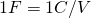In circuit diagrams a capacitor is noted by the following symbol: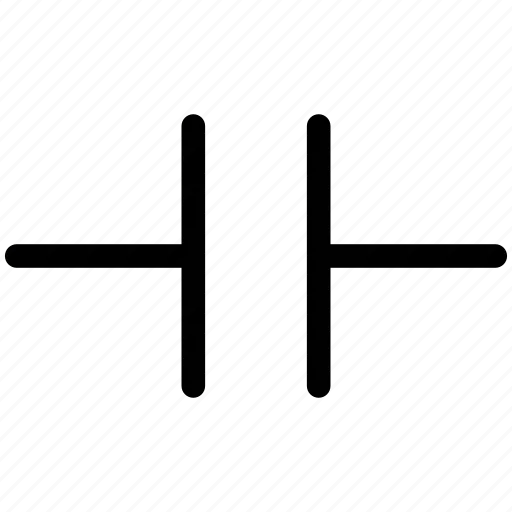Source

Capacitors are used a lot in electronic devices and those devices could not exist without them. It's very important to have some way of storing electrical energy.

## Calculating capacitance

As already mentioned before, the most common type of capacitor has two parallel conductive plates.

Let's say that each of them has an area A and that they have a distance d which is very small compared two the sizes of the plates.

The electric field of such a capacitor is mostly concentrated between those two plates.

There is still an electric field outside of the limits of the conductors, but when the distance is very small i's negligible.

That way the electric field between those two plates is uniform and the charge is uniformly distributed along the surfaces of the two facing sides of the conductors.

In an application that we covered previously during this series, we proved that the electric field can be calculated using the following equation: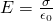where σ is of course the area density of charge.

The area density σ is calculated using:When having a uniform electric field between two parallel plates we have proven that:Using this equation we end up with this equation for potential difference: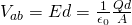Substituing Vab into the capacitance equation we get: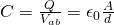where ε0, A and d are of course constant for any capacitor.

This proves that the capacitance is constant and doesn't depend on the charge of the capacitor. And so the capacitance is proportional to the Area and inverse proportional to the distance of the plates.

This last equation also tells us that capacitance can also be calculated in C^2/J, where 1J = 1 N*m.

That way ε0 can now also be described in: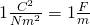This is what we will use for problems around capacitors...

Also remember that for all these calculations we specified "empty" space between the conductive plates. When having an insulator (or dielectric as we will call them later on) the calculations are of course different, but don't worry we will get into those capacitors some posts later on!

Intro

Physics Introduction -> what is physics?, Models, Measuring

Vector Math and Operations -> Vector mathematics and operations (actually mathematical analysis, but I don't got into that before-hand :P)

Classical Mechanics

Velocity and acceleration in a rectlinear motion -> velocity, accelaration and averages of those

Rectlinear motion with constant accelaration and free falling -> const accelaration motion and free fall

Rectlinear motion with variable acceleration and velocity relativity -> integrations to calculate pos and velocity, relative velocity

Rectlinear motion exercises -> examples and tasks in rectlinear motion

Position, velocity and acceleration vectors in a plane motion -> position, velocity and accelaration in plane motion

Projectile motion as a plane motion -> missile/bullet motion as a plane motion

Smooth Circular motion -> smooth circular motion theory

Plane motion exercises -> examples and tasks in plane motions

Force and Newton's first law -> force, 1st law

Mass and Newton's second law -> mass, 2nd law

Newton's 3rd law and mass vs weight -> mass vs weight, 3rd law, friction

Applying Newton's Laws -> free-body diagram, point equilibrium and 2nd law applications

Contact forces and friction -> contact force, friction

Dynamics of Circular motion -> circular motion dynamics, applications

Object equilibrium and 2nd law application examples -> examples of object equilibrium and 2nd law applications

Contact force and friction examples -> exercises in force and friction

Circular dynamic and vertical circle motion examples -> exercises in circular dynamics

Electromagnetism

Getting into Electromagnetism -> electromagnetim, electric charge, conductors, insulators, quantization

Coulomb's law with examples -> Coulomb's law, superposition principle, Coulomb constant, how to solve problems, examples

Electric fields and field lines -> Electric fields, Solving problems around Electric fields and field lines

Electric dipoles -> Electric dipole, torque, potential and field

Electric charge and field Exercises -> examples in electric charges and fields

Electric flux and Gauss's law -> Electric flux, Gauss's law

Applications of Gauss's law (part 1) -> applying Gauss's law, Gauss applications

Applications of Gauss's law (part 2) -> more Gauss applications

Electric flux exercises -> examples in electric flux and Gauss's law

Electric potential energy -> explanation of work-energy, electric potential energy

Calculating electric potentials -> more stuff about potential energy, potential, calculating potentials

Millikan's Oil Drop Experiment -> Millikan's experiment, electronvolt

Cathode ray tubes explained using electric potential -> cathode ray tube explanation

Electric potential exercises (part 1) -> applications of potential

Electric potential exercises (part 2) -> applications of potential gradient, advanced examples

And this is actually it for today!

Next time we will get into how we solve problems that contain Capacitors!

Bye!

H2
H3
H4
3 columns
2 columns
1 column